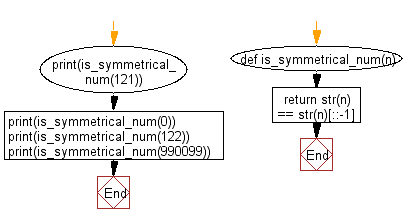﻿ Python: Test whether a given number is symmetrical or not - w3resource

# Python: Test whether a given number is symmetrical or not

## Python Basic - 1: Exercise-83 with Solution

Write a Python program to test whether a given number is symmetrical or not. A number is symmetrical when it is equal to its reverse.

For example- 121 is the symmetric number.

Sample Solution:

Python Code:

``````def is_symmetrical_num(n):
return str(n) == str(n)[::-1]
print(is_symmetrical_num(121))
print(is_symmetrical_num(0))
print(is_symmetrical_num(122))
print(is_symmetrical_num(990099))
``````

Sample Output:

```True
True
False
True
```

Pictorial Presentation:Flowchart:Python Code Editor:

Have another way to solve this solution? Contribute your code (and comments) through Disqus.

What is the difficulty level of this exercise?

Test your Programming skills with w3resource's quiz.

﻿